# UCVM Density Formula

We have reviewed how the density values are calculated in our tomography improved velocity models. We have determined that CVM-S4.26 calculated density from model Vs, and CCA06 provides density values in a data file. We reviewed algorithms to confirm that the same algorith is used to calculated density for both CVM-S4.6 and CCA06.

## Compare CCA06, CVM-S4.26 and CenCal Density to Nafe Drake Vs Density Relationship

If model uses the formula, we'd expect values to fall on a diagonal line in these plots.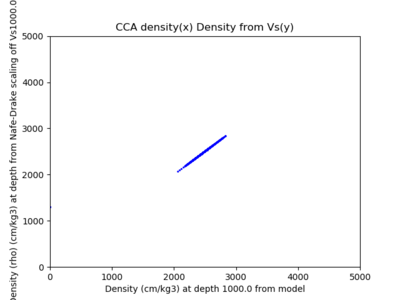CCA Density (rho) at Depth 1000m (Density in g/cm^3)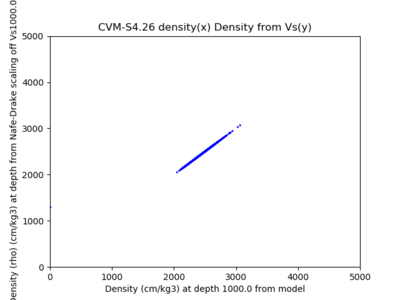CVM-S4.26 Density (rho) at Depth 1000m (Density in g/cm^3)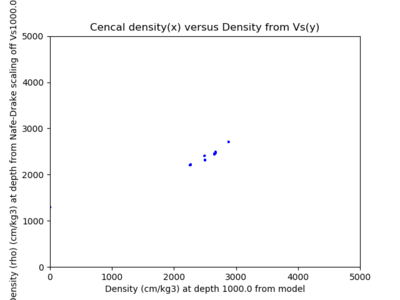Cencal Density (rho) at Depth 1000m

Looks like CCA06 and CVM-S4.26 use this Vs-based density scaling relationship, but CenCal and CVM-H do not.

## Compare CCA06, CVM-S4.26 and CenCal Density to Nafe Drake Vp Density Relationship

UCVM also provides an algorithm that defines a scaling relationship between Vp values and density values on a nafe drake density relationship. These plots show density values for selected points extracted from cvm-s4.26, cca06, and cencal, and cvm-h.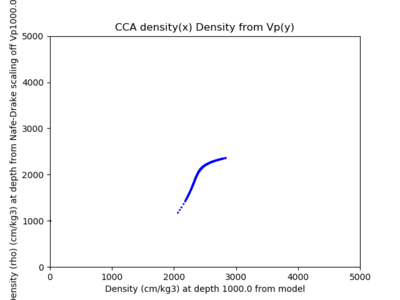CCA Density (rho) at Depth 1000m (Density in g/cm^3)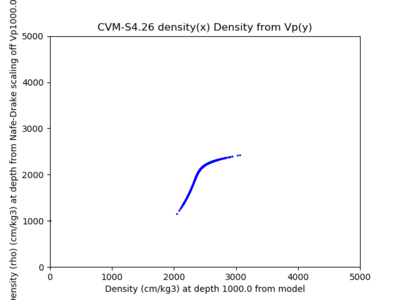CVM-S4.26 Density (rho) at Depth 1000m (Density in g/cm^3)

## Vs-based Density Formula used in CVM-S4.26 and CCA06

```
#
# * Calculates the density based off of Vs. Based on Nafe-Drake scaling relationship.
# *
# * @param vs The Vs value off which to scale.
# * @return Density, in g/m^3.
# */
def vs_2_density(vs):
# Density scaling parameters
p5 = -0.0024189659303912917
p4 = 0.015600987888334450
p3 = 0.051962399479341816
p2 = -0.51231936640441489
p1 = 1.2550758337054457
p0 = 1.2948318548300342

retVal = 0.0
vs = vs / 1000.0
retVal = p0 + p1 * vs + p2 * pow(vs, 2) + p3 * pow(vs, 3) + p4 * pow(vs, 4) + p5 * pow(vs, 5)
retVal = retVal * 1000
return retVal
```

## Vp-based Density Formula in UCVM

```# Density derived from Vp via Nafe-Drake curve, Brocher (2005) eqn 1. */
def vp_2_density(vp):
# Convert m to km
vp = vp * 0.001
rho = vp * (1.6612 - vp * (0.4721 - vp * (0.0671 - vp * (0.0043 - vp * 0.000106))))
if (rho < 1.0):
rho = 1.0
rho = rho * 1000.0;
return
```